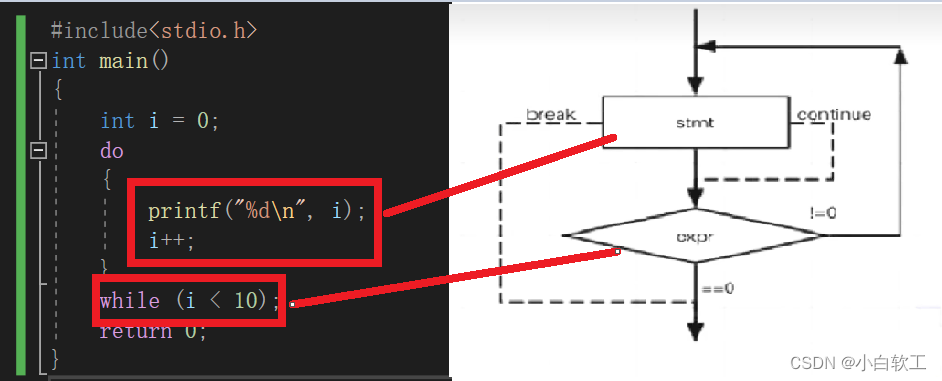# 【寒假自学C语言】 --4 三类循环语句总结及对比

1.不可在for循环内修改循环变量，否则程序失去控制。

3、特点：

## 1、示例：

用while循环打印1-9数字。

``````#include<stdio.h>
int main()
{
int i = 1;
while (i < 10)
{
printf("%d",i);
i++;
}
return 0;
}``````

## 2、流程图：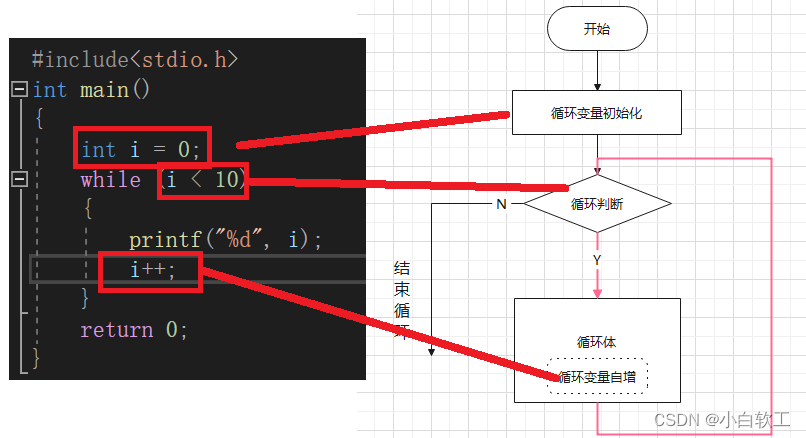通过图表以及代码对应关系来看，这样while循环的工作原理就会更加的清晰。

## 1、示例：

代码如下：

``````#include<stdio.h>
int main()
{
int  i, a, N;
a = 1;
scanf_s("%d,", &N);
for (i = 1; i < N; i++)
{
a = (a + 1) * 2;
}
printf("%d", a);
return 0;
}``````

## 2、流程图：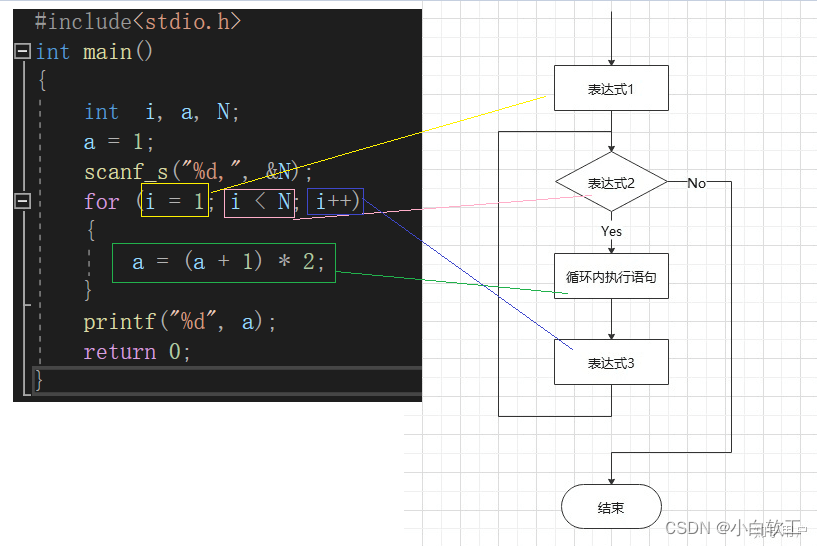## 3、注意事项：

### 1.不可在for循环内修改循环变量，否则程序失去控制。

``````#include<stdio.h>
int main()
{
int i = 0;
for (i = 0; i < 10; i++)
{
printf("%d\n", i);
}
return 0;
}
``````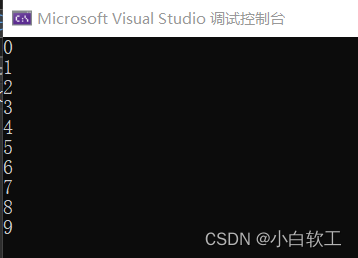``````#include<stdio.h>
int main()
{
int i = 0;
for (i =0; i < 10; i++)
{
if (i = 5)
printf("%d\n", i);
}
return 0;
}``````

编译结果如下所示：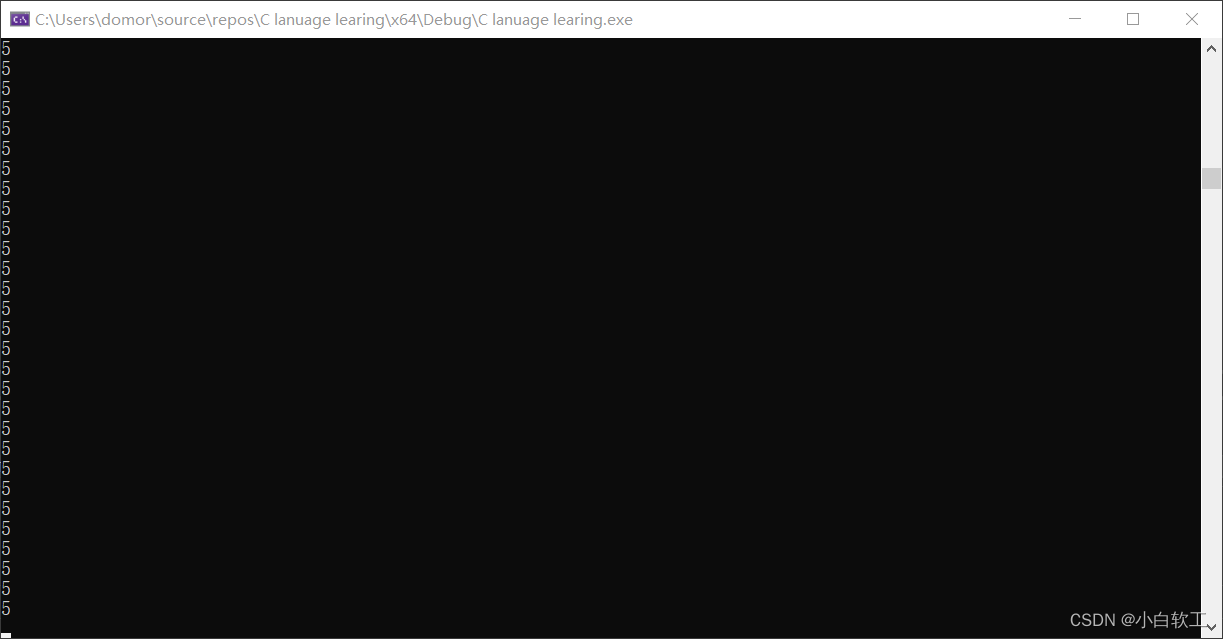### 2.建议for循环语句控制变量取值采用”前闭合后开区间“的写法。

这样做法看似多次一举，其实在项目较为复杂时候可以尽快判断出循环次数和其他重要信息。

## 1、示例：

``````#include<stdio.h>
int main()
{
int i = 0;
do
{
printf("%d\n", i);
i++;
}
while (i < 10);
return 0;
}``````

do下面执行语句必须加{}

## 2、流程图